# Derived Types C allows a number of derived

• Slides: 49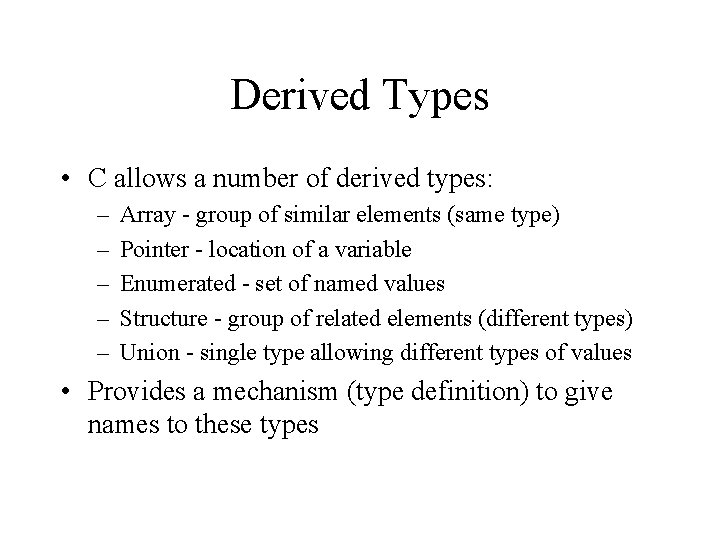Derived Types • C allows a number of derived types: – – – Array - group of similar elements (same type) Pointer - location of a variable Enumerated - set of named values Structure - group of related elements (different types) Union - single type allowing different types of values • Provides a mechanism (type definition) to give names to these typesOutline • Type Definition • Enumerated Type – definition, representation • Structured Type – – – definition, initialization, assignment, comparison passing as parameter (value, ref), return value pointer selection operator arrays within structures (nested structures) arrays of structures (tables) • reading group, writing group, insert, remove, sort, print • Union Type – definition, use within structures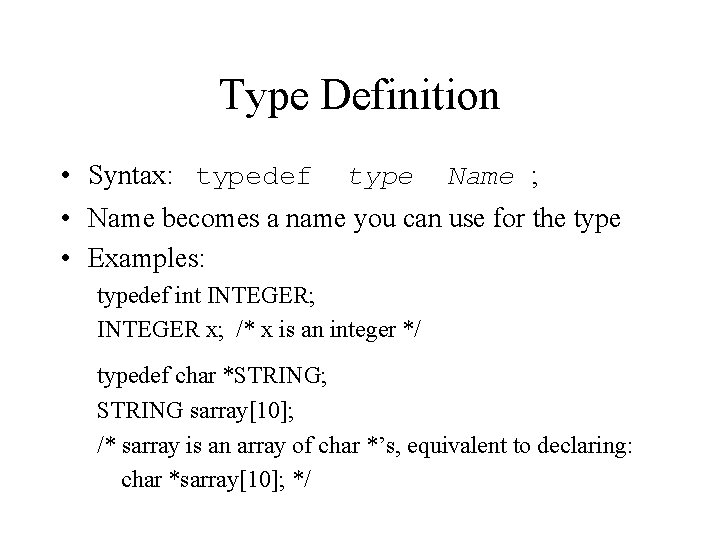Type Definition • Syntax: typedef type Name ; • Name becomes a name you can use for the type • Examples: typedef int INTEGER; INTEGER x; /* x is an integer */ typedef char *STRING; STRING sarray; /* sarray is an array of char *’s, equivalent to declaring: char *sarray; */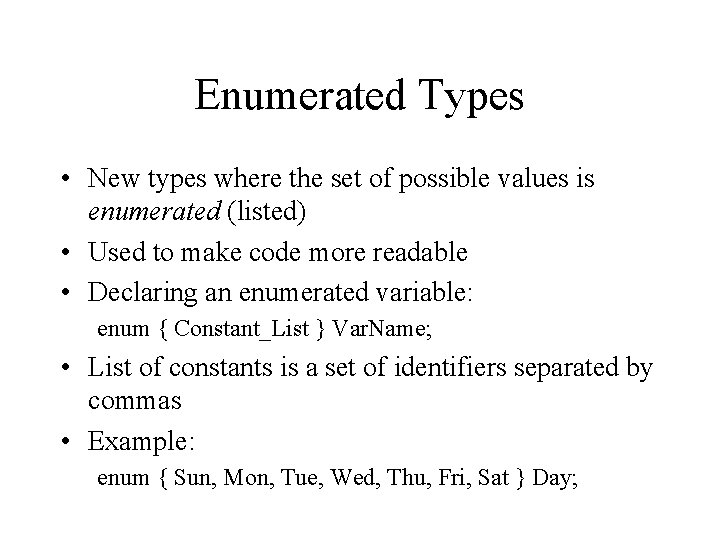Enumerated Types • New types where the set of possible values is enumerated (listed) • Used to make code more readable • Declaring an enumerated variable: enum { Constant_List } Var. Name; • List of constants is a set of identifiers separated by commas • Example: enum { Sun, Mon, Tue, Wed, Thu, Fri, Sat } Day;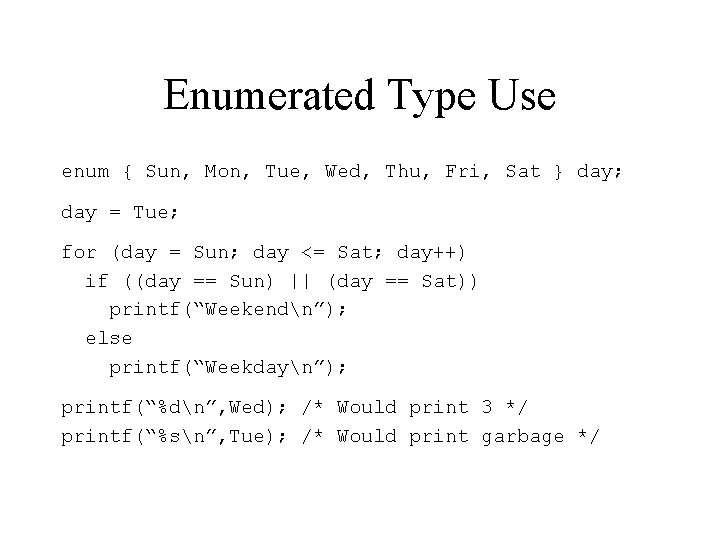Enumerated Type Use enum { Sun, Mon, Tue, Wed, Thu, Fri, Sat } day; day = Tue; for (day = Sun; day <= Sat; day++) if ((day == Sun) || (day == Sat)) printf(“Weekendn”); else printf(“Weekdayn”); printf(“%dn”, Wed); /* Would print 3 */ printf(“%sn”, Tue); /* Would print garbage */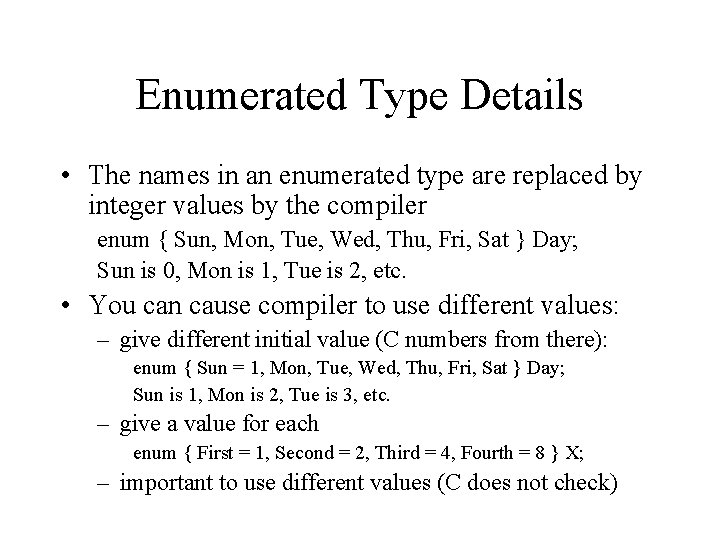Enumerated Type Details • The names in an enumerated type are replaced by integer values by the compiler enum { Sun, Mon, Tue, Wed, Thu, Fri, Sat } Day; Sun is 0, Mon is 1, Tue is 2, etc. • You can cause compiler to use different values: – give different initial value (C numbers from there): enum { Sun = 1, Mon, Tue, Wed, Thu, Fri, Sat } Day; Sun is 1, Mon is 2, Tue is 3, etc. – give a value for each enum { First = 1, Second = 2, Third = 4, Fourth = 8 } X; – important to use different values (C does not check)Building Enumerated Types • Declaring type: (outside of function) Tag: enum Tag { Const. List }; Defined type: typedef enum { Const. List } Type. Name; Examples: enum Day. Type 1 { Sun, Mon, Tue, Wed, Thu, Fri, Sat }; typedef enum { Sun, Mon, Tue, Wed, Thu, Fri, Sat } Day. Type 2; • Variable declarations: Tag: enum Tag Var. Name; Defined type: Type. Name Var. Name; Examples: enum Day. Type 1 Day. Var 1; Day. Type 2 Day. Var 2;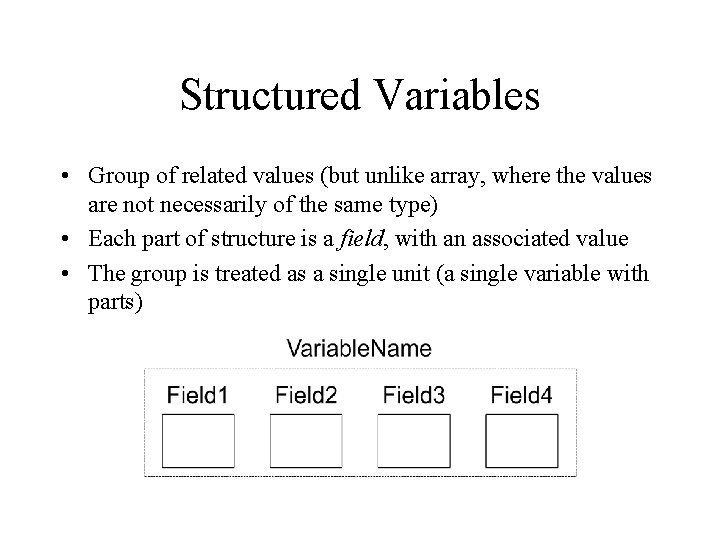Structured Variables • Group of related values (but unlike array, where the values are not necessarily of the same type) • Each part of structure is a field, with an associated value • The group is treated as a single unit (a single variable with parts)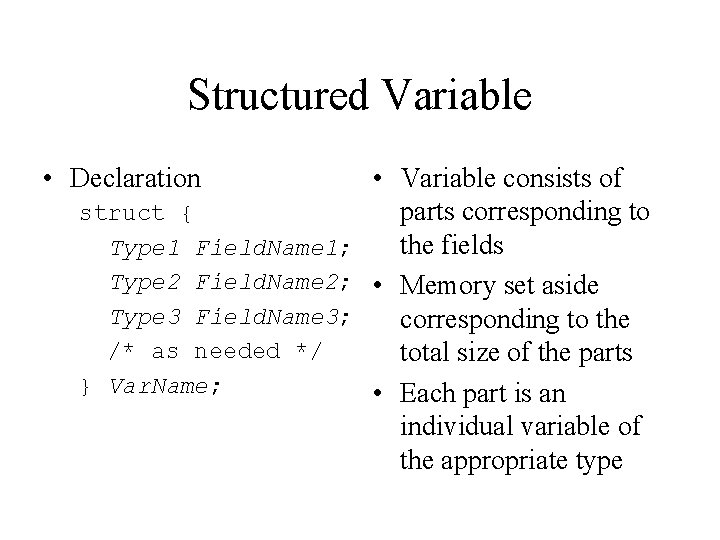Structured Variable • Declaration • Variable consists of struct { parts corresponding to the fields Type 1 Field. Name 1; Type 2 Field. Name 2; • Memory set aside Type 3 Field. Name 3; corresponding to the /* as needed */ total size of the parts } Var. Name; • Each part is an individual variable of the appropriate typeStructure Types Tag declaration: struct Tag. Name { Type 1 Field 1; Type 2 Field 2; Type 3 Field 3; /* any more */ }; Variable declaration: struct Tag. Name Var. N; Type definition: typedef struct { Type 1 Field 1; Type 2 Field 2; Type 3 Field 3; /* any more */ } Type. Name; Variable declaration: Type. Name Var. N;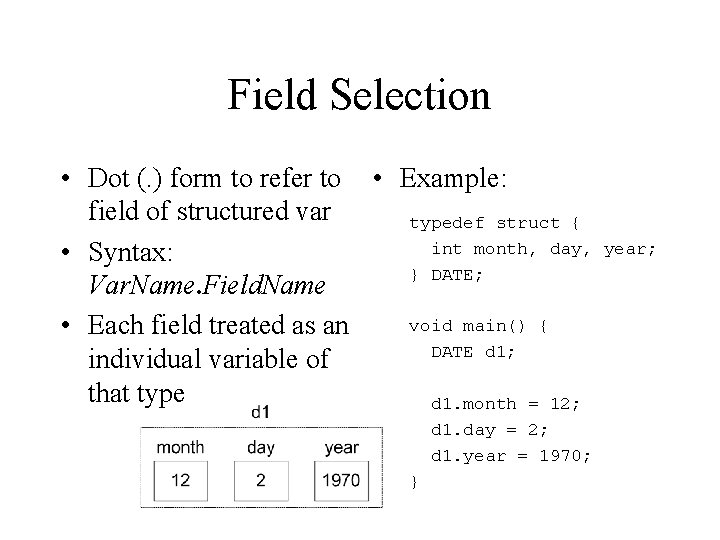Field Selection • Dot (. ) form to refer to • Example: field of structured var typedef struct { int month, day, • Syntax: } DATE; Var. Name. Field. Name void main() { • Each field treated as an DATE d 1; individual variable of that type d 1. month = 12; d 1. day = 2; d 1. year = 1970; } year;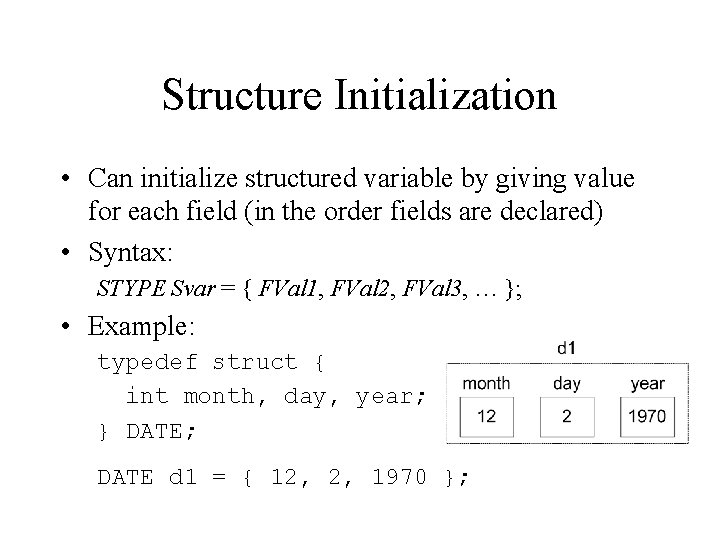Structure Initialization • Can initialize structured variable by giving value for each field (in the order fields are declared) • Syntax: STYPE Svar = { FVal 1, FVal 2, FVal 3, … }; • Example: typedef struct { int month, day, year; } DATE; DATE d 1 = { 12, 2, 1970 };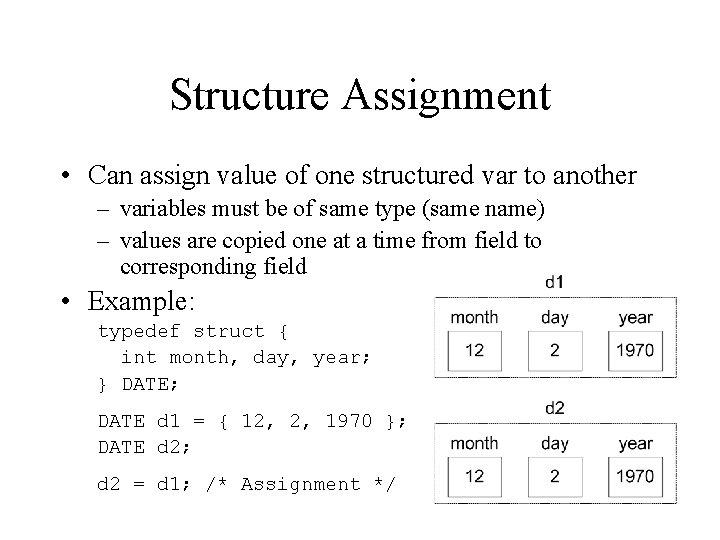Structure Assignment • Can assign value of one structured var to another – variables must be of same type (same name) – values are copied one at a time from field to corresponding field • Example: typedef struct { int month, day, year; } DATE; DATE d 1 = { 12, 2, 1970 }; DATE d 2; d 2 = d 1; /* Assignment */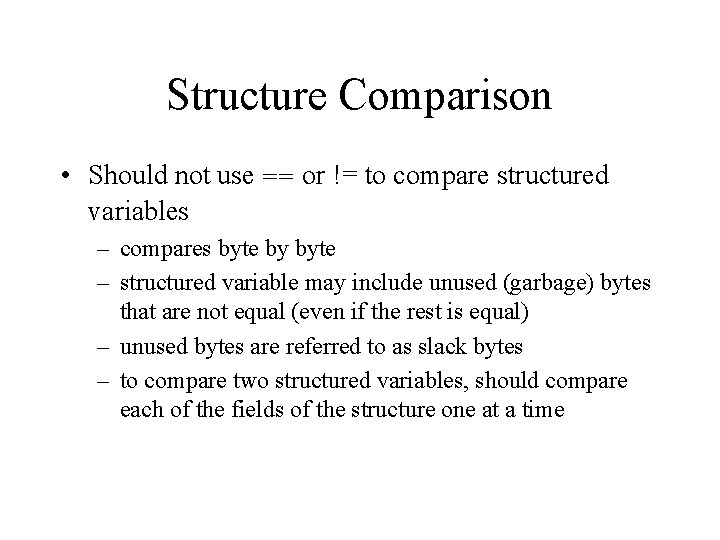Structure Comparison • Should not use == or != to compare structured variables – compares byte by byte – structured variable may include unused (garbage) bytes that are not equal (even if the rest is equal) – unused bytes are referred to as slack bytes – to compare two structured variables, should compare each of the fields of the structure one at a time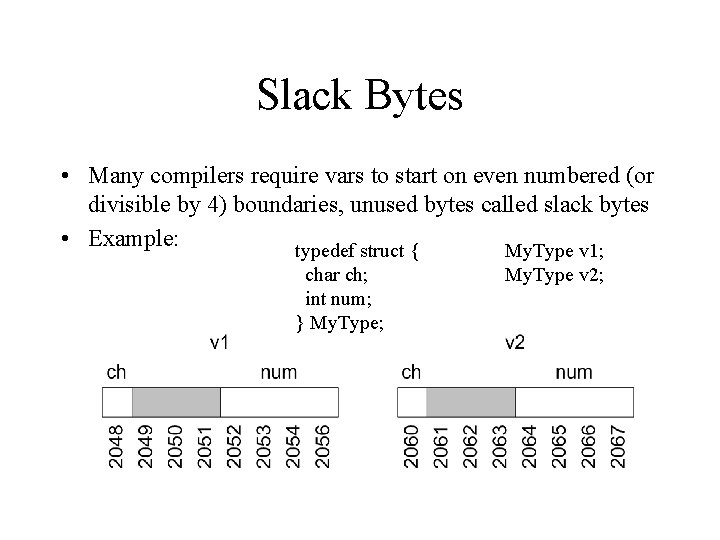Slack Bytes • Many compilers require vars to start on even numbered (or divisible by 4) boundaries, unused bytes called slack bytes • Example: typedef struct { My. Type v 1; char ch; int num; } My. Type; My. Type v 2;Structure Example #include <stdio. h> printf(”Enter: n”); printf(" ID#: "); scanf("%d", &s 1. id); printf(" GPA: "); scanf("%f", &s 1. gpa); printf(" Class: "); scanf(" %c", &s 1. class); typedef struct { int id; float gpa; char class; } Student; void main() { Student s 1; printf(”S#%d (%c) gpa = %. 3 fn", s 1. id, s 1. class, s 1. gpa); }Passing Structures as Parameters • A field of a structure may be passed as a parameter (of the type of that field) • An advantage of structures is that the group of values may be passed as a single structure parameter (rather than passing individual vars) • Structures can be used as – value parameter: fields copied (as in assignment stmt) – reference parameter: address of structure passed – return value (resulting structure used in statement) -not all versions of C allow structured return value – best to use type-defined structuresStructure as Value Parameter #include <stdio. h> typedef struct { int id; float gpa; char class; } Student; void print. S(Student s) { /* Struc. Param. named s created, fields of arg (s 1) copied to s */ printf("S#%d (%c) gpa = %. 3 fn", s. id, s. class, s. gpa); } void main() { Student s 1; s 1 = read. S(); print. S(s 1); }Structure as Return Value typedef struct { int id; float gpa; char class; } Student; void main() { Student s 1; Student read. S() { Student s; /* local */ printf(”Enter: n"); printf(" ID#: "); scanf("%d", &s. id); printf(" GPA: "); scanf("%f", &s. gpa); printf(" Class: "); scanf(" %c", &s. class); s 1 = read. S(); print. S(s 1); } return s; /* local as return val */ }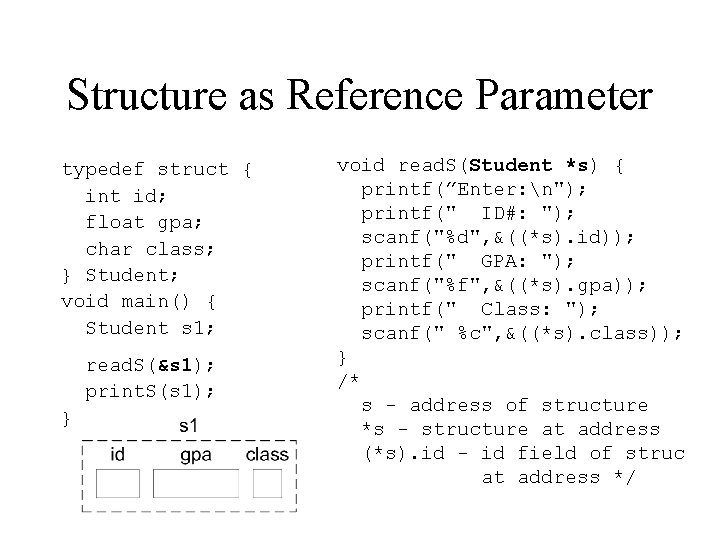Structure as Reference Parameter typedef struct { int id; float gpa; char class; } Student; void main() { Student s 1; read. S(&s 1); print. S(s 1); } void read. S(Student *s) { printf(”Enter: n"); printf(" ID#: "); scanf("%d", &((*s). id)); printf(" GPA: "); scanf("%f", &((*s). gpa)); printf(" Class: "); scanf(" %c", &((*s). class)); } /* s - address of structure *s - structure at address (*s). id - id field of struc at address */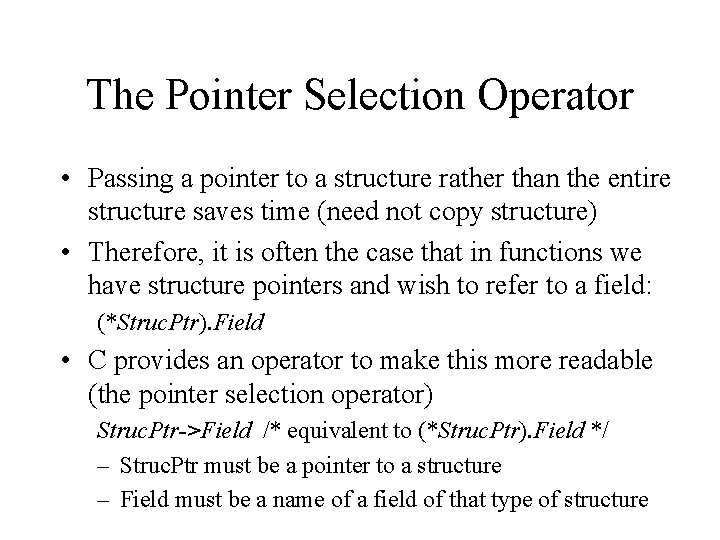The Pointer Selection Operator • Passing a pointer to a structure rather than the entire structure saves time (need not copy structure) • Therefore, it is often the case that in functions we have structure pointers and wish to refer to a field: (*Struc. Ptr). Field • C provides an operator to make this more readable (the pointer selection operator) Struc. Ptr->Field /* equivalent to (*Struc. Ptr). Field */ – Struc. Ptr must be a pointer to a structure – Field must be a name of a field of that type of structure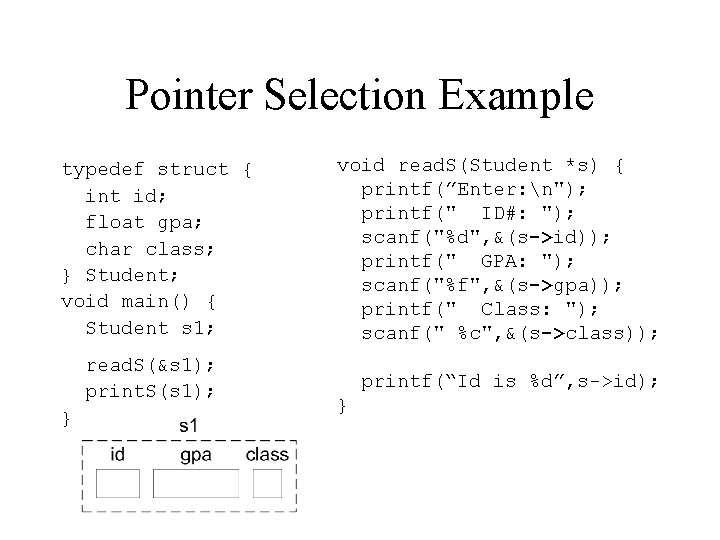Pointer Selection Example typedef struct { int id; float gpa; char class; } Student; void main() { Student s 1; read. S(&s 1); print. S(s 1); } void read. S(Student *s) { printf(”Enter: n"); printf(" ID#: "); scanf("%d", &(s->id)); printf(" GPA: "); scanf("%f", &(s->gpa)); printf(" Class: "); scanf(" %c", &(s->class)); printf(“Id is %d”, s->id); }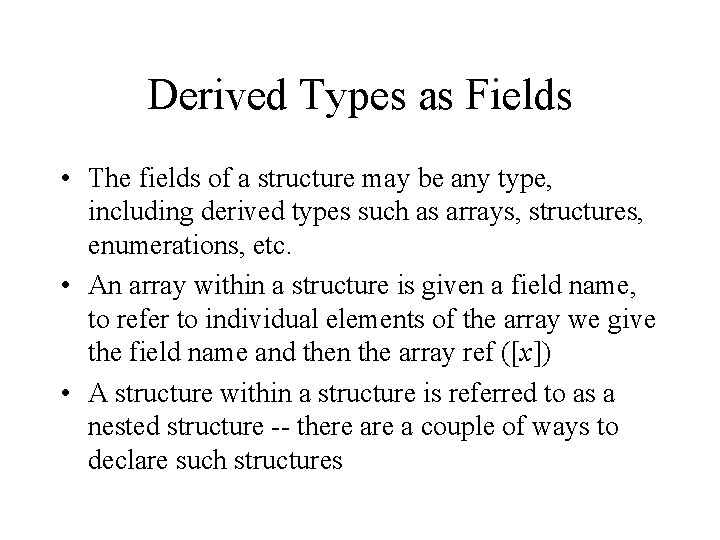Derived Types as Fields • The fields of a structure may be any type, including derived types such as arrays, structures, enumerations, etc. • An array within a structure is given a field name, to refer to individual elements of the array we give the field name and then the array ref ([x]) • A structure within a structure is referred to as a nested structure -- there a couple of ways to declare such structures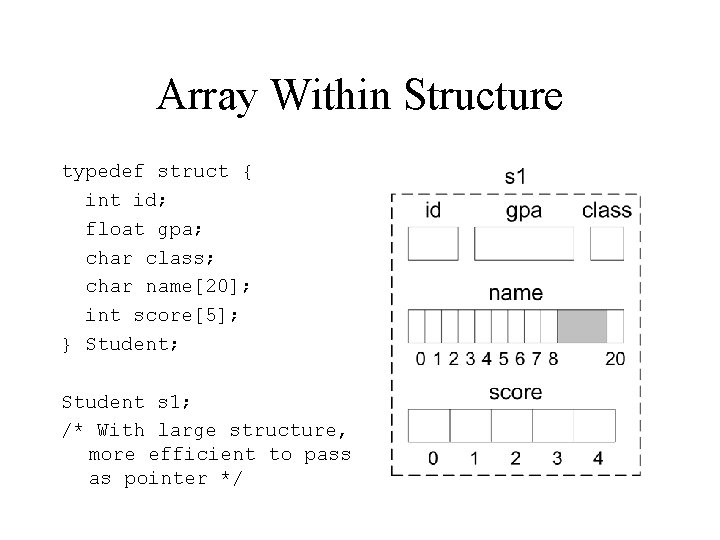Array Within Structure typedef struct { int id; float gpa; char class; char name; int score; } Student; Student s 1; /* With large structure, more efficient to pass as pointer */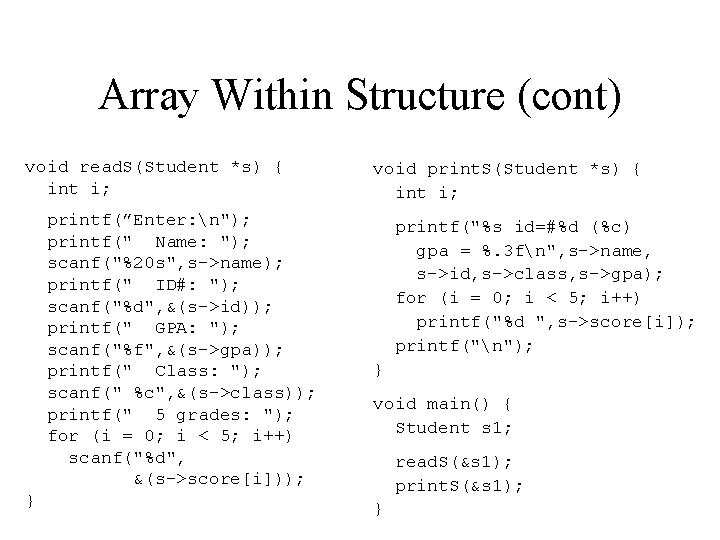Array Within Structure (cont) void read. S(Student *s) { int i; printf(”Enter: n"); printf(" Name: "); scanf("%20 s", s->name); printf(" ID#: "); scanf("%d", &(s->id)); printf(" GPA: "); scanf("%f", &(s->gpa)); printf(" Class: "); scanf(" %c", &(s->class)); printf(" 5 grades: "); for (i = 0; i < 5; i++) scanf("%d", &(s->score[i])); } void print. S(Student *s) { int i; printf("%s id=#%d (%c) gpa = %. 3 fn", s->name, s->id, s->class, s->gpa); for (i = 0; i < 5; i++) printf("%d ", s->score[i]); printf("n"); } void main() { Student s 1; read. S(&s 1); print. S(&s 1); }Nested Structure One mechanism, declare nested • Fields of V: structure directly within type V. A /* int field */ definition: V. D /* structure field */ typedef struct { int A; struct { char B; float C; } D; /* struc field */ } My. Type; My. Type V; V. D. B /* char field */ V. D. C /* float field */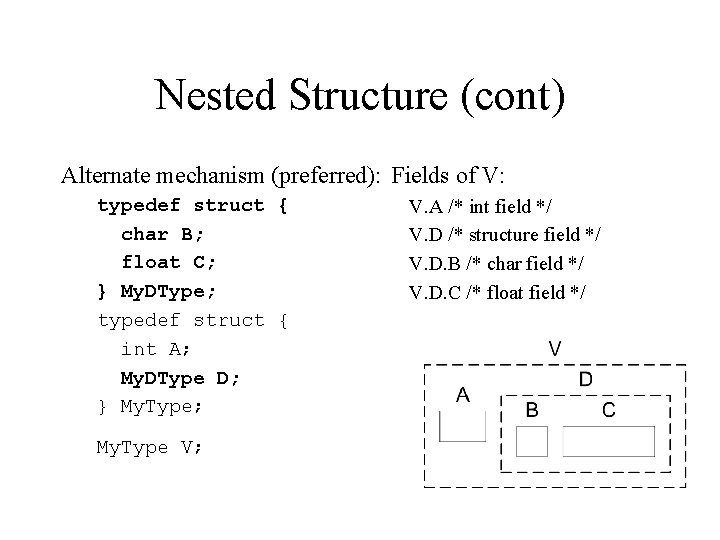Nested Structure (cont) Alternate mechanism (preferred): Fields of V: typedef struct { char B; float C; } My. DType; typedef struct { int A; My. DType D; } My. Type; My. Type V; V. A /* int field */ V. D /* structure field */ V. D. B /* char field */ V. D. C /* float field */Initializing Nested Structures • To initialize a nested structure we give as the value of the structure a list of values for the substructure: Struc. Type V = { Field 1 Val, Field 2 Val, Field 3 Val, … } where Field. XVal is an item of the form { Sub. Field 1 Val, Sub. Field 2 Val, Sub. Field 3 Val, … } if Field X is a structured field • Previous example (My. Type) My. Type V = { 43, { ‘A’, 3. 5 } };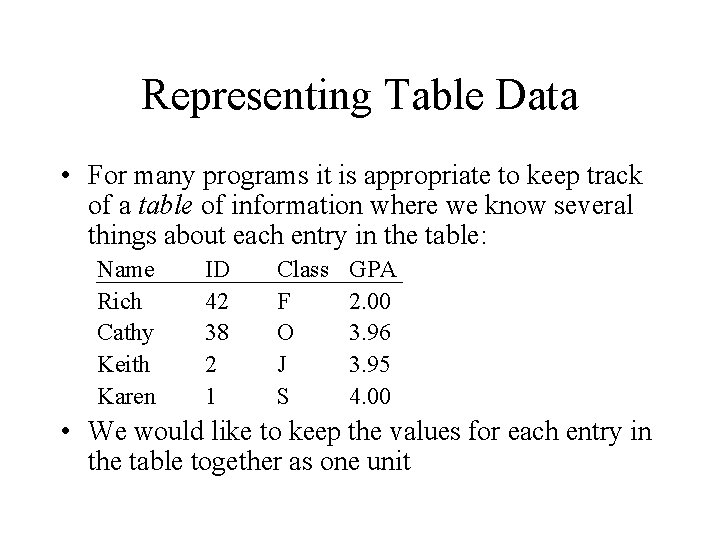Representing Table Data • For many programs it is appropriate to keep track of a table of information where we know several things about each entry in the table: Name Rich Cathy Keith Karen ID 42 38 2 1 Class F O J S GPA 2. 00 3. 96 3. 95 4. 00 • We would like to keep the values for each entry in the table together as one unitTable: Array of Structures • One mechanism for representing a table of information is to use an array where each member of the array is a structure representing the info about a line of the table: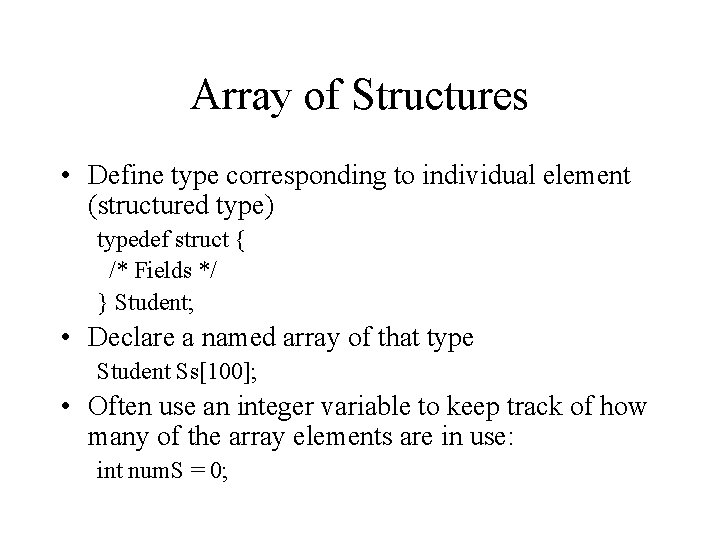Array of Structures • Define type corresponding to individual element (structured type) typedef struct { /* Fields */ } Student; • Declare a named array of that type Student Ss; • Often use an integer variable to keep track of how many of the array elements are in use: int num. S = 0;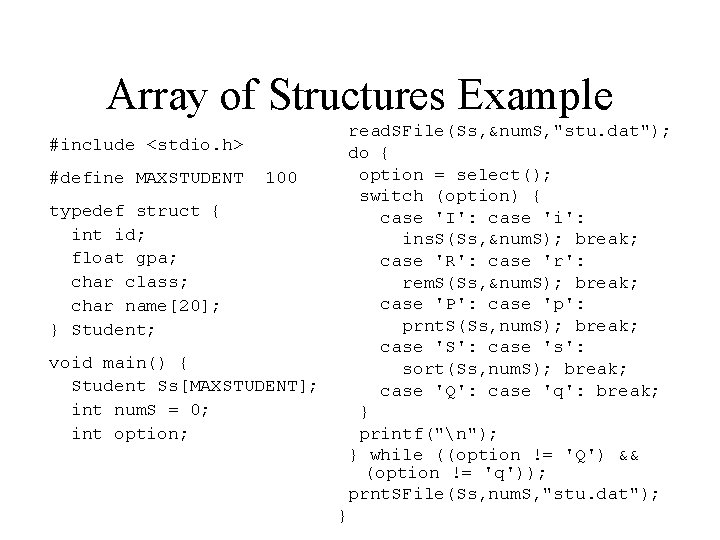Array of Structures Example read. SFile(Ss, &num. S, "stu. dat"); do { option = select(); switch (option) { case 'I': case 'i': ins. S(Ss, &num. S); break; case 'R': case 'r': rem. S(Ss, &num. S); break; case 'P': case 'p': prnt. S(Ss, num. S); break; case 'S': case 's': sort(Ss, num. S); break; case 'Q': case 'q': break; } printf("n"); } while ((option != 'Q') && (option != 'q')); prnt. SFile(Ss, num. S, "stu. dat"); #include <stdio. h> #define MAXSTUDENT 100 typedef struct { int id; float gpa; char class; char name; } Student; void main() { Student Ss[MAXSTUDENT]; int num. S = 0; int option; }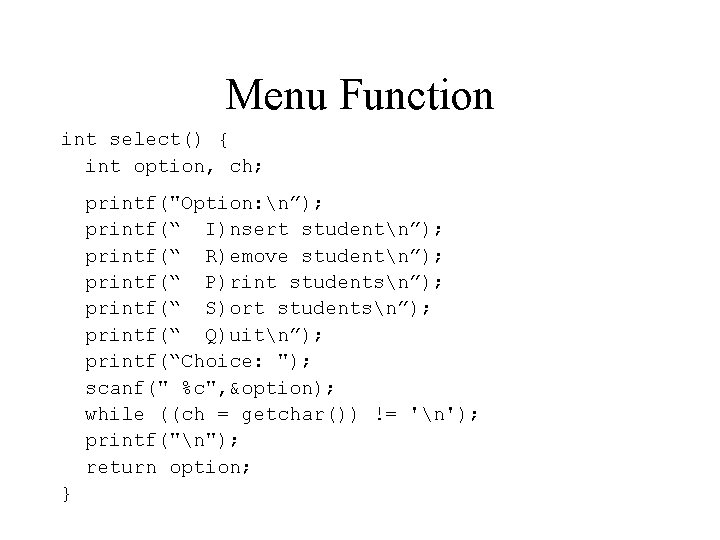Menu Function int select() { int option, ch; printf("Option: n”); printf(“ I)nsert studentn”); printf(“ R)emove studentn”); printf(“ P)rint studentsn”); printf(“ S)ort studentsn”); printf(“ Q)uitn”); printf(“Choice: "); scanf(" %c", &option); while ((ch = getchar()) != 'n'); printf("n"); return option; }Initializing Array • Often the data in the table is entered over multiple uses of the program – At end of program save file of info, read file at start • Write the data for each table item out in format: – ints, floats, chars separated by whitespace – strings on separate lines (followed by newline) • Read the data, one entry at a time: – use fgets to read strings (terminated by newline)Saving Table Info to File void prnt. SFile(Student Ss[], int num. S, char *fname) { FILE *outs; int J; if ((outs = fopen(fname, "w")) == NULL) { printf("Unable to open file %sn", fname); exit(-1); } fprintf(outs, ”%dn”, num. S); /* Save # of students */ for (J = 0; J < num. S; J++) /* Print student J */ fprintf(outs, ”%c %d %fn%sn“, Ss[J]. class, Ss[J]. id, Ss[J]. gpa, Ss[J]. name); fclose(outs); }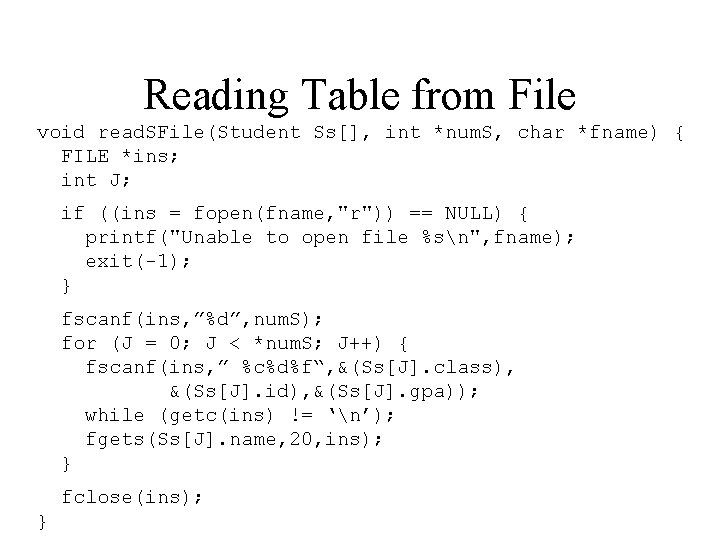Reading Table from File void read. SFile(Student Ss[], int *num. S, char *fname) { FILE *ins; int J; if ((ins = fopen(fname, "r")) == NULL) { printf("Unable to open file %sn", fname); exit(-1); } fscanf(ins, ”%d”, num. S); for (J = 0; J < *num. S; J++) { fscanf(ins, ” %c%d%f“, &(Ss[J]. class), &(Ss[J]. id), &(Ss[J]. gpa)); while (getc(ins) != ‘n’); fgets(Ss[J]. name, 20, ins); } fclose(ins); }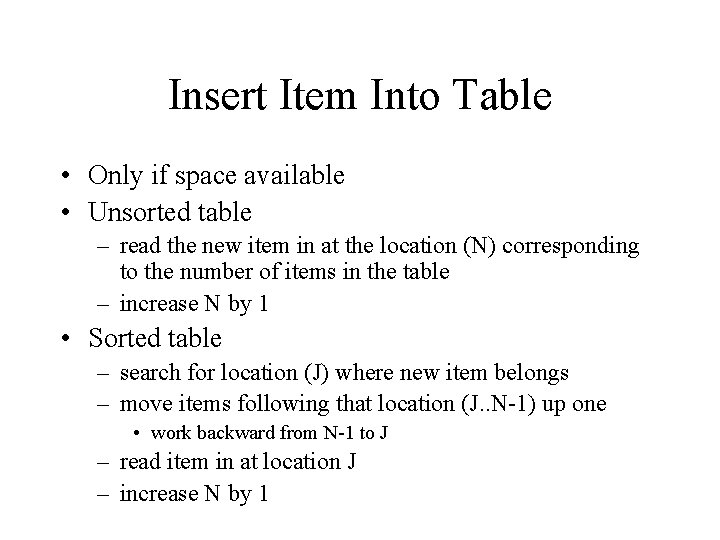Insert Item Into Table • Only if space available • Unsorted table – read the new item in at the location (N) corresponding to the number of items in the table – increase N by 1 • Sorted table – search for location (J) where new item belongs – move items following that location (J. . N-1) up one • work backward from N-1 to J – read item in at location J – increase N by 1Inserting Student void read. S(Student *s) { int I; printf(”Enter: n"); printf(" Name: "); fgets(s->name, 19, stdin); I = strlen(s->name) - 1; if (s->name[I] == ‘n’) s->name[I] = ‘’; printf(" ID#: "); scanf("%d", &(s->id)); printf(" GPA: "); scanf("%f", &(s->gpa)); printf(" Class: "); scanf(" %c", &(s->class)); } void ins. S(Student Ss[], int *num. S) { if (*num. S < MAXSTUDENT) { read. S(&(Ss[*num. S])); *num. S += 1; } else printf("Sorry, cannot add any more students no room!n"); }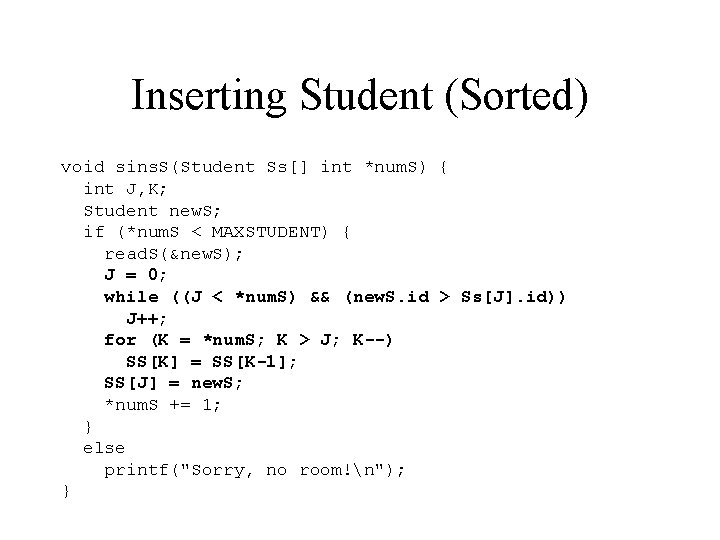Inserting Student (Sorted) void sins. S(Student Ss[] int *num. S) { int J, K; Student new. S; if (*num. S < MAXSTUDENT) { read. S(&new. S); J = 0; while ((J < *num. S) && (new. S. id > Ss[J]. id)) J++; for (K = *num. S; K > J; K--) SS[K] = SS[K-1]; SS[J] = new. S; *num. S += 1; } else printf("Sorry, no room!n"); }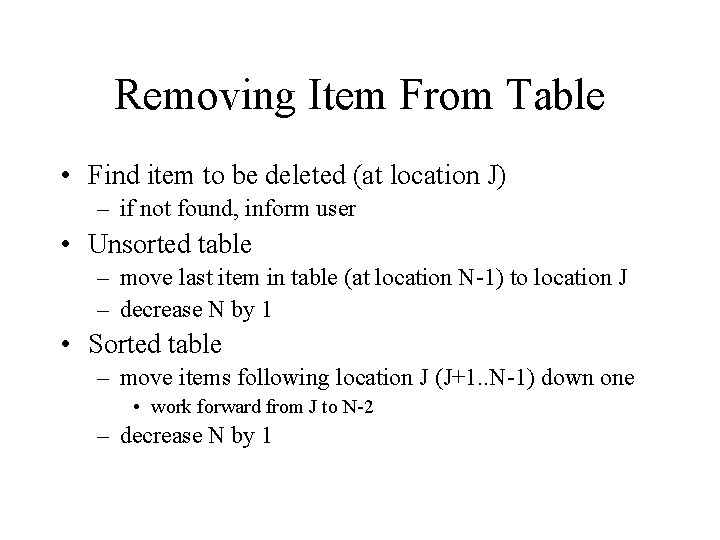Removing Item From Table • Find item to be deleted (at location J) – if not found, inform user • Unsorted table – move last item in table (at location N-1) to location J – decrease N by 1 • Sorted table – move items following location J (J+1. . N-1) down one • work forward from J to N-2 – decrease N by 1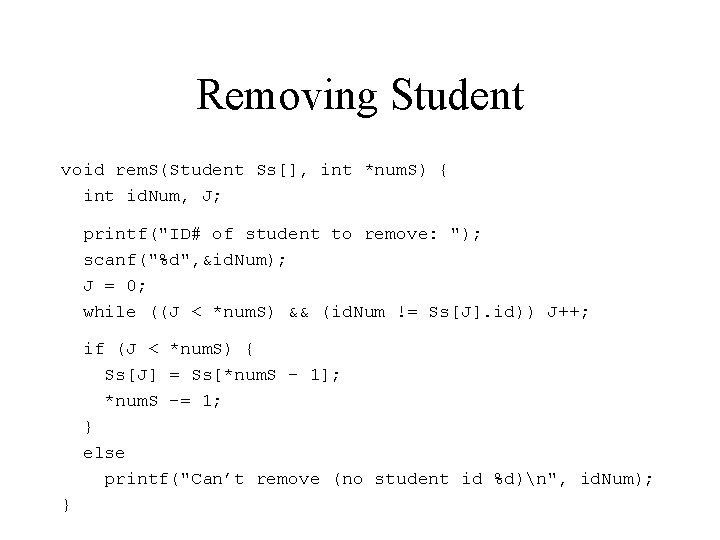Removing Student void rem. S(Student Ss[], int *num. S) { int id. Num, J; printf("ID# of student to remove: "); scanf("%d", &id. Num); J = 0; while ((J < *num. S) && (id. Num != Ss[J]. id)) J++; if (J < *num. S) { Ss[J] = Ss[*num. S - 1]; *num. S -= 1; } else printf("Can’t remove (no student id %d)n", id. Num); }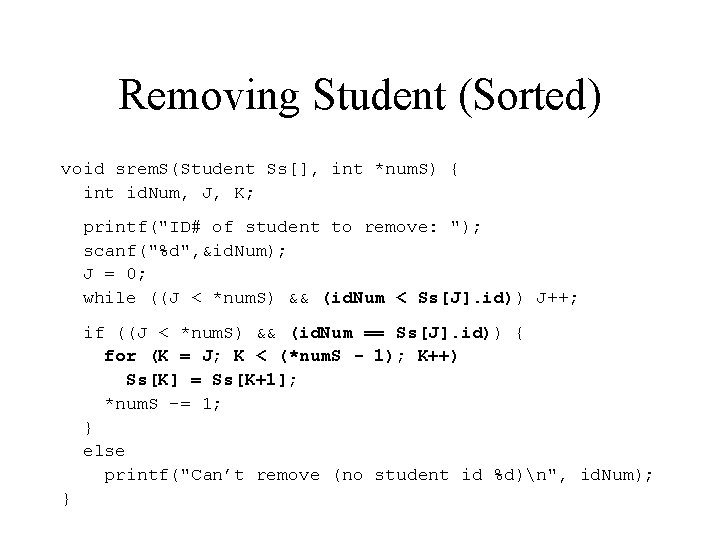Removing Student (Sorted) void srem. S(Student Ss[], int *num. S) { int id. Num, J, K; printf("ID# of student to remove: "); scanf("%d", &id. Num); J = 0; while ((J < *num. S) && (id. Num < Ss[J]. id)) J++; if ((J < *num. S) && (id. Num == Ss[J]. id)) { for (K = J; K < (*num. S - 1); K++) Ss[K] = Ss[K+1]; *num. S -= 1; } else printf("Can’t remove (no student id %d)n", id. Num); }Printing Students void prnt. S(Student Ss[], int num. S) { int J; printf(“Student ID# C GPAn”); printf(“----------------n”); for (J = 0; J < numstudents; J++) printf("%-20 s %3 d %c %5. 3 fn", Ss[J]. name, Ss[J]. id, Ss[J]. class, Ss[J]. gpa); }Sorting Table • Pick an appropriate sorting method – selection sort is appropriate if the structures are large – insert sort if you expect the elements to be nearly sorted • Determine the field or fields from the structures that will compared to determine which elements are small – determine an appropriate test for that field (if string use strcmp, for most other fields use <)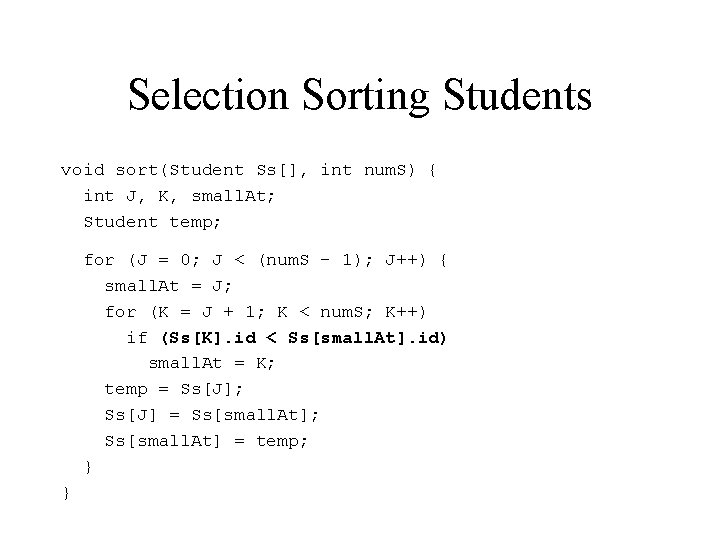Selection Sorting Students void sort(Student Ss[], int num. S) { int J, K, small. At; Student temp; for (J = 0; J < (num. S - 1); J++) { small. At = J; for (K = J + 1; K < num. S; K++) if (Ss[K]. id < Ss[small. At]. id) small. At = K; temp = Ss[J]; Ss[J] = Ss[small. At]; Ss[small. At] = temp; } }Union Variables • Union vars used to store different value types • Syntax: union { Type 1 FName 1; Type 2 FName 2; /* as needed */ } Var. Name; • Example: union { char A; int B; float C; } V; • V has enough space to hold the largest of the three fields (in this case C) • Can use each of the different fields: V. A = ‘a’; V. B = 42; V. C = 2. 5; • But each of the fields uses the same space (so storing in V. B changes the bits stored in V. A)Union Types Tag Syntax: union Tag { Type 1 FName 1; Type 2 FName 2; /* as needed */ }; union Tag Vname; Example: union Utype 1 { char A; int B; float C; }; union Utype 1 V; Defined Type Syntax: typedef union { Type 1 FName 1; Type 2 FName 2; /* as needed */ } TName; TName Vname; Example: typedef union { char A; int B; float C; } Utype 2; Utype 2 V;Unions in Structures • Union types often used in structures where fields of structure depend item represented • Example: – Seniors - number of credits to graduation – Juniors - upper-division GPA – Freshmen - character value indicating if student is instate or out-of-state • Often use another value in structure to determine which applies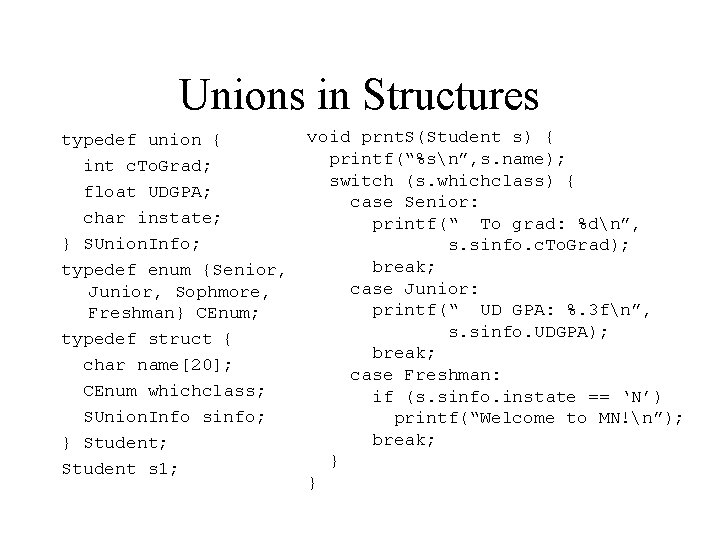Unions in Structures typedef union { int c. To. Grad; float UDGPA; char instate; } SUnion. Info; typedef enum {Senior, Junior, Sophmore, Freshman} CEnum; typedef struct { char name; CEnum whichclass; SUnion. Info sinfo; } Student; Student s 1; void prnt. S(Student s) { printf(“%sn”, s. name); switch (s. whichclass) { case Senior: printf(“ To grad: %dn”, s. sinfo. c. To. Grad); break; case Junior: printf(“ UD GPA: %. 3 fn”, s. sinfo. UDGPA); break; case Freshman: if (s. sinfo. instate == ‘N’) printf(“Welcome to MN!n”); break; } }